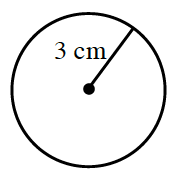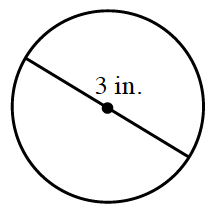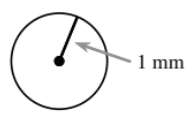### Home > CC2MN > Chapter 10 > Lesson 10.2.2 > Problem10-46

10-46.

Read the Math Notes box for this lesson and then find the length of the radius and diameter of each of the following circles.

The radius of a circle is a line segment from its center to any point on the circle. The diameter of a circle is a line segment going through the center and ending on opposite sides of the circle.

1.Radius: $3$ cm
Diameter: $6$ cm

1.How is the information for this circle different than part (a)?

Radius: $1.5$ in.
Diameter: $3$ in.

1.Use the same method as part (a).